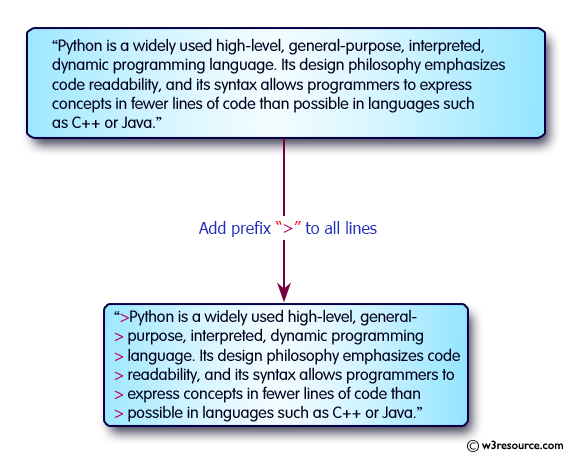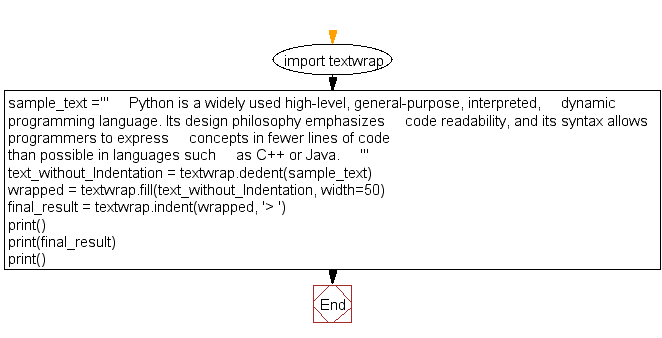﻿ Python: Add a prefix text to all of the lines in a string - w3resource

# Python: Add a prefix text to all of the lines in a string

## Python String: Exercise-28 with Solution

Write a Python program to add prefix text to all of the lines in a string.Sample Solution:-

Python Code:

``````import textwrap
sample_text ='''
Python is a widely used high-level, general-purpose, interpreted,
dynamic programming language. Its design philosophy emphasizes
code readability, and its syntax allows programmers to express
concepts in fewer lines of code than possible in languages such
as C++ or Java.
'''
text_without_Indentation = textwrap.dedent(sample_text)
wrapped = textwrap.fill(text_without_Indentation, width=50)
#wrapped += '\n\nSecond paragraph after a blank line.'
final_result = textwrap.indent(wrapped, '> ')
print()
print(final_result)
print()
```
```

Sample Output:

```>  Python is a widely used high-level, general-
> purpose, interpreted, dynamic programming
> language. Its design philosophy emphasizes code
> readability, and its syntax allows programmers to
> express concepts in fewer lines of code than
> possible in languages such as C++ or Java.
```

Flowchart:Python Code Editor:

Have another way to solve this solution? Contribute your code (and comments) through Disqus.

What is the difficulty level of this exercise?

Test your Programming skills with w3resource's quiz.

﻿

## Python: Tips of the Day

Creates a list of elements, grouped based on the position in the original lists:

Example:

```def tips_zip(*args, fill_value=None):
max_length = max([len(lst) for lst in args])
result = []
for i in range(max_length):
result.append([
args[k][i] if i < len(args[k]) else fillvalue for k in range(len(args))
])
return result
print(tips_zip(['a', 'b'], [1, 2], [True, False]))
print(tips_zip(['a'], [1, 2], [True, False]))
print(tips_zip(['a'], [1, 2], [True, False], fill_value = '1'))
```

Output:

```[['a', 1, True], ['b', 2, False]]
[['a', 1, True], [None, 2, False]]
[['a', 1, True], ['1', 2, False]]
```